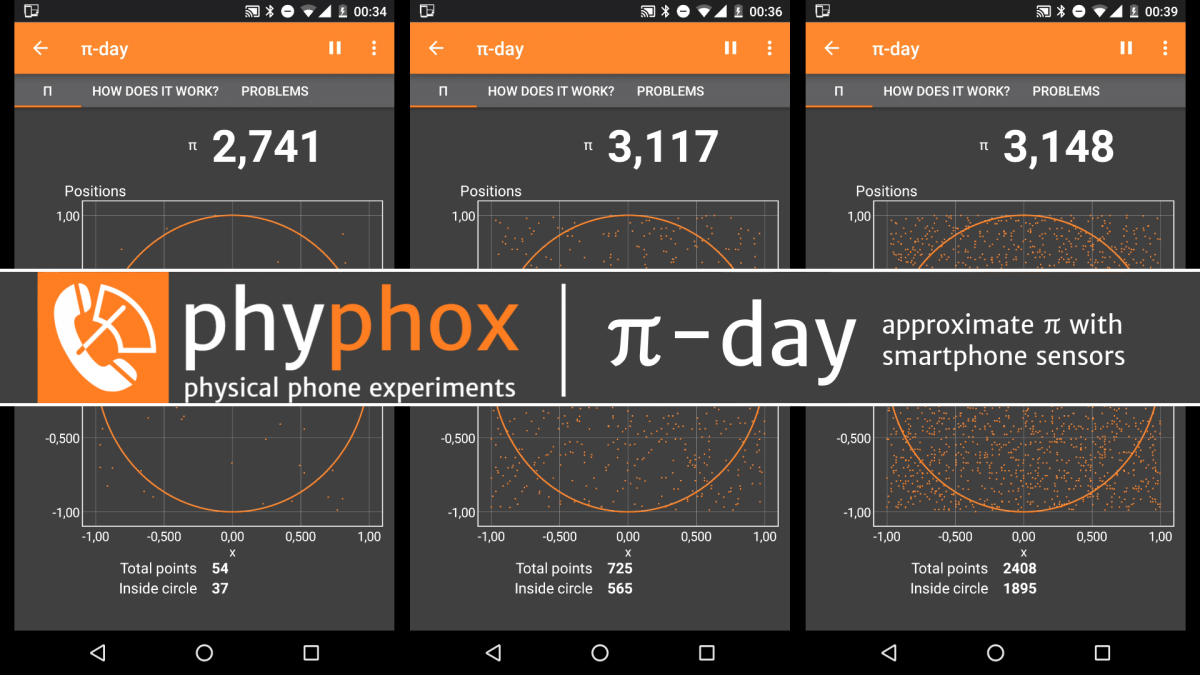# π-dayLet’s celebrate π-day by approximating π with smartphone sensors! All you need is phyphox and this experiment:

## How does it work?

This experiment generates random numbers from the noise of the accelerometer in your phone. These random numbers are used to create a point at a random location (x,y) within a square, ranging from -1 to +1 on the x and on the y axis.

You will notice that there is a circle drawn into this square range. This is a circle with radius 1. By calculating the distance r² = x² + y² of a point at (x,y), we can tell if it is within the circle (r < 1) or if it is on the outside (r > 1).

The probability for a point to be inside is proportional to the ratio of the area of the circle to the area of the square. The area of the square is 4 as each side has the length 2. The area of the circle is π, because its radius is 1 (the area of a circle with radius r is πr²). Therefore the probability for a point to be inside the circle is π/4.

Putting it all together, all we need to do is calculating the ratio of points inside the circle to the total number of points. π is four times this ratio.

## Problems

This method requires equally distributed random numbers, which we generate from the decimal part of the sensor readings. This leads to the following possible problems:

• While the first digit will stabilize very fast, it will take somewhat longer for the next one to stabilize. If you want a good result for the last digit shown in this experiment, expect to acquire 10000 or even 100000 values before getting a satisfactory result.
• Your smartphone needs to be resting to get an approximately unbiased distribution.
• Depending on the accelerometer in your phone or the way it handles the data, you may still not get unbiased and equally distributed data. In this case you will notice patterns in the way the points are scattered in the graph and your result may be systematically off.

Contact: contact@phyphox.org
Phyphox has been created at the 2nd Institute of Physics of the RWTH Aachen University.